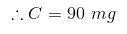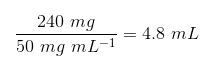top of page## Tutorial 2 - How do I calculate my nic?

Pre-Note: I recommend the use of protective equipment such as latex gloves when undertaking mixing of e-liquids. Furthermore, I recommend the use of a clean and dedicated mixing area to prevent cross contaminations, with adequate spill control and clean up equipment.
I personally do not condone the use of nic base above concentrations of 100mg/mL under any circumstances, and pure (99.9%) nic should never be purchased for the safety of yourself, those around you, and those having to package/courier the base materials.

I tend to trust my own math more than using other sources, so do this to make sure I am on point. This will not go into detail about calculating PG/VG ratios, or concentration percentage calculations (these are seperate tutorials). It is solely based on calculating your end nic strength.

So... how much of my nic solution do I need to add to any given volume to get it to my desired strength?
Great question.

This is a relatively simple method, and can easily be done with the use of a simple calculator without much knowledge of mathematical theory (but it helps to understand the reasoning).

Basically, when you are adding nic to an e-liquid, you already know all of the variables required to calculate the final volume (in millilitres) of your nic base to get your desired end strength.

1) The concentration of your nic base solution (in milligrams per millilitre) - if you don't know this, you definitely should NOT be using your nic base.
2) The end concentration you want your liquid to be (also in milligrams per millilitre). - typically 3, 6, 12, 18, or 24 milligrams per millilitre, these strengths can also be known as percentages as such - 0.3% 0.6% 1.2% 1.8% 2.4%. This tutorial will use 3mg/mL, but this value can simply be subbed out in the below equations.
3) The total volume of your end product e-liquid. For the purposes of this tutorial, I will be using the standard small bottle size of 30mL. Again, this can simply be subbed out for larger (or smaller) volumes if you want to make a 1mL or 1000mL bottle.

So, you know the above, but what you don't know yet - how much volume of my nicotine base do I need exactly to reach my end desired result?....

Well, this is a simple two step calculation.
Bear with me, it is REALLY simple. Once you know the equation, you can apply it for any volume, of any strength, using any concentrate of nic base solution.

To construct our equation we will denote our known and unknown values as follows:
A = Final nic strength required in milligrams per millilitre - this is the end result you want, like when you buy a liquid at 12mg/mL.
B = Final volume of solution - this is the end volume you want to end up with, like 30mL in a bottle of the same size.
C = Total nic required in your mix, measured in milligrams only - unlike A and B, you don't know this yet.

So, the variable C is what we need to find in the first step of this calculation, as follows:

EQUATION 1

Now, lets plug some values into this to make it make a bit more sense.

I want my end strength to be 3mg/mL, in an end volume of 30mL of e-liquid.

So we have:

And we can see that there is a total of 90mg of nic required in the 30mL of solution.
If you are a math nerd, you can see how the units cancel each other perfectly, and thus we know it is correct.

CONGRATULATIONS!
Step one is complete.

Now, we know the following values:
C = Total nic required in your mix, measured in milligrams.
D = The concentration of your nic base solution, measured in milligrams per millilitre. As per point 2 above, you already know this - its written on the bottle......

What we don't know is:
E = The total volume of your nic base required to reach the desired end strength, measured in millilitres.

We can construct the second equation using the outcome of the first equation above, as follows:

EQUATION 2

Now, as I need 90mg of nic in my solution, and I have a 36mg/mL base, I know that:

And plugging these into equation 2 we have:

BOOM!

We now know that we need a total of 2.5mL of our nic base added to our mix to make our desired strength of 3mg/mL.
Again, we know it is sound as all of the units cancel out appropriately.

You can simply substitute any of the values as needed to get your required end result, doesn't matter if you have 100mg/mL nic base, are mixing 1litre of e-liquid, or want a final strength of 24mg/mL.
The above method will allow you to calculate any of these in a simple two steps.

EXAMPLE 2
Lets try it with a different nic base, for a different volume and different end strength:

STEP 1

and thus:
STEP 2

Happy days!

I hope that this helps anyone aiming to run through these calculations. After a couple of runs, it becomes second nature to do these calculations.bottom of page